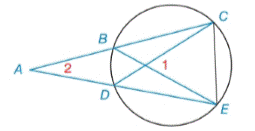Chapter 6.2, Problem 17E### Elementary Geometry for College St...

6th Edition
Daniel C. Alexander + 1 other
ISBN: 9781285195698

#### Solutions

Chapter
Section### Elementary Geometry for College St...

6th Edition
Daniel C. Alexander + 1 other
ISBN: 9781285195698
Textbook Problem
6 views

# Given: m ∠ 1 = 62 ° m ∠ 2 = 26 ° Find: m C E ⌢ and m B D ⌢Exercises 16, 17

To determine

To find:

mCE and mBD when m1=62°,m2=26°

Explanation

mCE=xmBD=y

Theorem:

The measure of an angle formed by two chords that intersect within a circle is one-half the sum of the measures of the arcs intercepted by the angle and its vertical angle.

mCE and mBD when m1=62°,m2=26°

By Theorem

m1=12(mCE+mBD)62°=12(x+y)x+y=2(62°)x+y=124°

Theorem:

The measure of an angle formed when two secants intersect at a point outside the circle is one-half the difference of the measures of the two intercepted arc

### Still sussing out bartleby?

Check out a sample textbook solution.

See a sample solution

#### The Solution to Your Study Problems

Bartleby provides explanations to thousands of textbook problems written by our experts, many with advanced degrees!

Get Started

#### Evaluate the integrals in Problems 1-26.

Mathematical Applications for the Management, Life, and Social Sciences

#### For 0

Study Guide for Stewart's Multivariable Calculus, 8th

#### The area of the shaded region is given by:

Study Guide for Stewart's Single Variable Calculus: Early Transcendentals, 8th